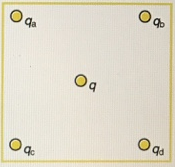# Problem: Four charges are arranged at the corners of a square, as depicted in the figure.(a) Using the symmetry of the arrangement, determine the direction of the electric field at the center of the square due to the four charges at the corners, given that qa = qb = -3.4 μC and qc = qd = 3.4 μC. Multiple Choice:1) Out of the screen2) Down 3) Right 4) Left5) Into the screen6) Up(b) Calculate the magnitude of the electric field, in newtons per coulomb, at the center of the square (the location of q), given that the square is 5.5 cm on a side. Numeric: A numeric value is expected and not an expression.I|E| = _________

###### FREE Expert Solution

Electric field:

$\overline{){\mathbf{E}}{\mathbf{=}}{\mathbf{k}}\frac{\mathbf{q}}{{\mathbf{r}}^{\mathbf{2}}}}$

q is the charge feeling the second charge feeling the force.

88% (191 ratings)###### Problem Details

Four charges are arranged at the corners of a square, as depicted in the figure.(a) Using the symmetry of the arrangement, determine the direction of the electric field at the center of the square due to the four charges at the corners, given that qa = qb = -3.4 μC and qc = q= 3.4 μC.

Multiple Choice:

1) Out of the screen

2) Down

3) Right

4) Left

5) Into the screen

6) Up

(b) Calculate the magnitude of the electric field, in newtons per coulomb, at the center of the square (the location of q), given that the square is 5.5 cm on a side.

Numeric: A numeric value is expected and not an expression.

I|E| = _________